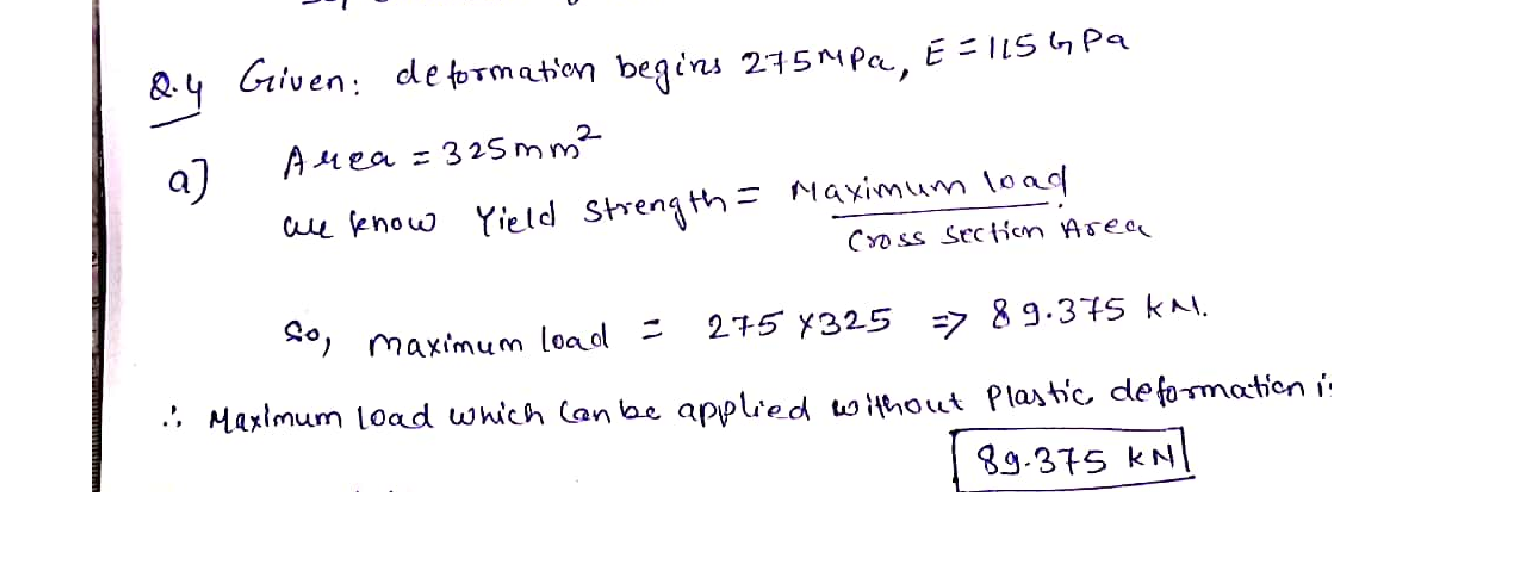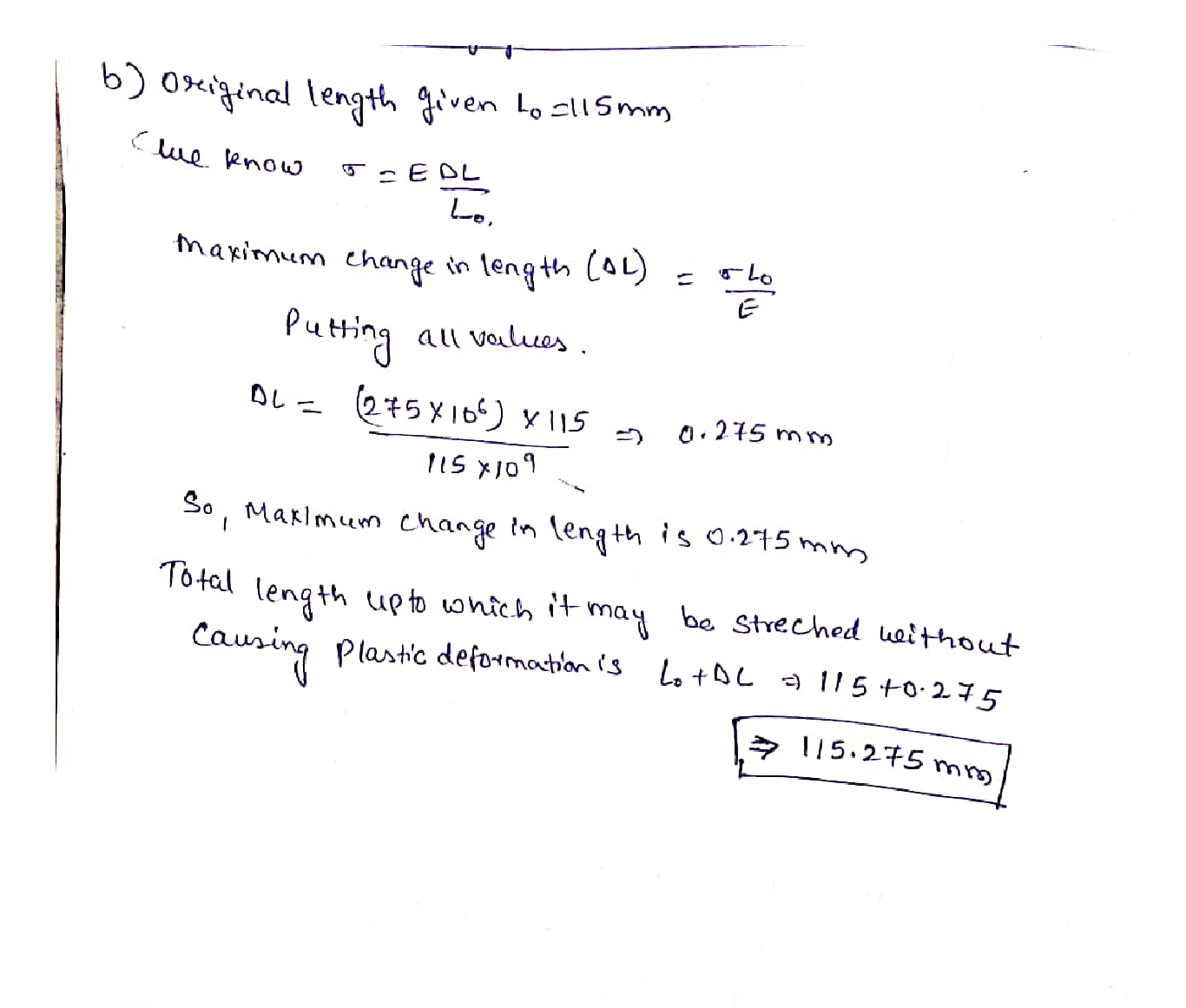Question

Composite Material

Questión 4:For a bronze alloy, the stress at which plastic deformation begins is 275 MPa and the modulus of elasticity is 115 GPa. (6 marks – 3 marks each part)

(a) What is the maximum load that may be applied to a specimen with a cross-sectional area of 325 mm without plastic deformation?

(b) If the original specimen length is 115 mm, what is the maximum length to which it may be stretched without causing plastic deformation?Verified### Question 30359Composite Material

Questión 4:For a bronze alloy, the stress at which plastic deformation begins is 275 MPa and the modulus of elasticity is 115 GPa. (6 marks – 3 marks each part)
(a) What is the maximum load that may be applied to a specimen with a cross-sectional area of 325 mm without plastic deformation?
(b) If the original specimen length is 115 mm, what is the maximum length to which it may be stretched without causing plastic deformation?

### Question 30358Composite Material

Suppose you want to connect a remote speaker to your stereo. It needs to be 100 inches away.You are using copper wire. What should be the diameter of the wire if the resistance of each wire must be less than 0.10 Q? (3 marks)

### Question 30357Composite Material

Draw stress- strain curve by hand and clearly show the following points on your curve. (2marks)
4. Uniform elastic deformation region
2. Yield strength point
3. Young's modulus (Modulus of elasticity)
1. Ultimate tensile strength

### Question 30356Composite Material

Do research, provide and explain two advantages and two disadvantages of using composite materials with examples. Make sure to provide references and each point should not be more than 2 lines. (4 marks)

### Question 21691Composite Material

16-109. Member AB has the angular motions shown.Determine the angular velocity and angular acceleration of members CB and DC.

### Question 21690Composite Material

16-107. At a given instant the roller A on the bar has the velocity and acceleration shown. Determine the velocity and acceleration of the roller B, and the bar's angular velocity and angular acceleration at this instant.

### Question 21689Composite Material

*16-88. If bar AB has an angular velocity w AB = 6rad/s determine the velocity of the slider block C at the instant shown.

### Question 21688Composite Material

16-74. The epicyclic gear train consists of the sun gear A which is in mesh with the planet gear B. This gear has an inner hub C which is fixed to B and in mesh with the fixed ring gear R. If the connecting link DE pinned to B and C is rotating at wnE = 18 rad/s about the pin at E, determine the angular velocities of the planet and sun gears.

### Question 21687Composite Material

*16-68. Knowing that angular velocity of link AB is WAB = 4 rad/s, determine the velocity of the collar at C and the angular velocity of link CB at the instant shown. Link CB is horizontal at this instant.

### Question 21686Composite Material

16-67. Determine the velocity of point A on the rim of the gear at the instant shown.

### Submit query

Getting answers to your urgent problems is simple. Submit your query in the given box and get answers Instantly.

### Submit a new QuerySuccess

Assignment is successfully created